4 Citations   Views   Downloads

## Introduction

In 1969, Gregory and Karney published a book of test matrices (Gregory & Karney, 1969). They stated that “In order to test the accuracy of computer programs for solving numerical problems, one needs numerical examples with known solutions. The aim of this monograph is to provide the reader with suitable examples for testing algorithms for finding the inverses, eigenvalues, and eigenvectors of matrix.” At that time it was common for journal papers to be devoted to introducing and analyzing a particular test matrix or class of matrices, examples being the papers of Clement (1959) (in the first issue of SIAM Review), Pei (1962) (occupying just a quarter of a page), and Gear (1969).

Today, test matrices remain of great interest, but not for the same reasons as fifty years ago. Testing accuracy using problems with known solutions is less common because a reference solution correct to machine precision can usually be computed at higher precision without difficulty. The main uses of test matrices nowadays are for exploring the behavior of mathematical quantities (such as eigenvalue bounds) and for measuring the performance of one or more algorithms with respect to accuracy, stability, convergence rate, speed, or robustness.

Various collections of matrices have been made available in software. As well as giving easy access to matrices these collections have the advantage of facilitating reproducibility of experiments (Donoho & Stodden, 2015), whether by the same researcher months later or by different researchers.

An early collection of parametrizable matrices was given by Higham (1991) and made available in MATLAB form. The collection was later extended and distributed as a MATLAB toolbox (Higham, 1995). Many of the matrices in the toolbox were subsequently incorporated into the MATLAB gallery function. Marques, Vömel, Demmel, and Parlett (Marques et al., 2008) present test matrices for tridiagonal eigenvalue problems (already recognized as important by Gregory and Karney, who devoted the last chapter of their book to such matrices). The Harwell–Boeing collection of sparse matrices (Duff, Grimes & Lewis, 1989) has been widely used, and is incorporated in the University of Florida Sparse Matrix Collection1 (Davis & Hu, 2011), which contains over 2700 matrices from practical applications, including standard and generalized eigenvalue problems from Bai et al. (1997). Among other MATLAB toolboxes we mention the CONTEST toolbox (Taylor & Higham, 2009), which produces adjacency matrices describing random networks, and the NLEVP collection of nonlinear eigenvalue problems (Betcke et al., 2013).

The purpose of this work is to provide a test matrix collection for Julia (Bezanson et al., 2014; Bezanson et al., 2012), a new dynamic programming language for technical computing. The collection, called Matrix Depot, exploits Julia’s multiple dispatch features to enable all matrices to be accessed by one simple interface. Moreover, Matrix Depot is extensible. Users can add matrices from the University of Florida Sparse Matrix Collection and Matrix Market; they can code new matrix generators and incorporate them into Matrix Depot; and they can define new groups of matrices that give easy access to subsets of matrices. The parametrized matrices can be generated in any appropriate numeric data type, such as

• floating-point types Float16 (half precision: 16 bits), Float32 (single precision: 32 bits), and Float64 (double precision: 64 bits);

• integer types Int32 (signed 32-bit integers), UInt32 (unsigned 32-bit integers), Int64 (signed 64-bit integers), and UInt64 (unsigned 64-bit integers);

• Complex, where the real and imaginary parts are of any Real type (the same for both);

• Rational (ratio of integers); and

• arbitrary precision type BigFloat (with default precision 256 bits), which uses the GNU MPFR Library (Fousse et al., 2007).

This paper is organized as follows. We start by giving a brief demonstration of Matrix Depot in ‘A Taste of Matrix Depot.’ Then we explain the design and implementation of Matrix Depot in ‘Package Design and Implementation,’ giving details on how multiple dispatch is exploited; how the collection is stored, accessed, and documented; and how it can be extended. In ‘The Matrices’ we describe the two classes of matrices in Matrix Depot: parametrized test matrices and real-life sparse matrix data. Concluding remarks are given in the final section.

## A Taste of Matrix Depot

To download Matrix Depot, in a Julia REPL (read-eval-print loop) run the command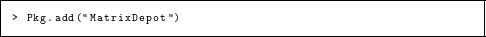Then import Matrix Depot into the local scope.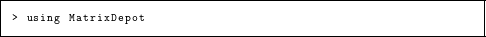Now the package is ready to be used. First, we find out what matrices are in Matrix Depot.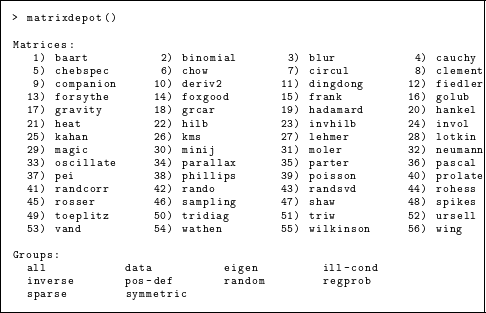All the matrices and groups in the collection are shown. It is also possible to obtain just the list of matrix names.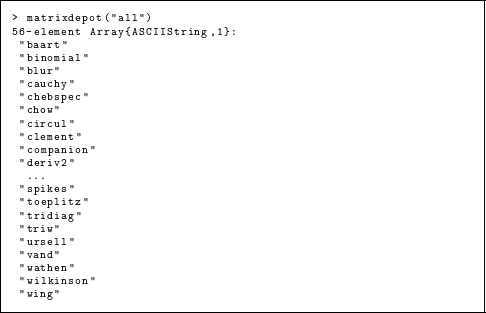Here, “ ...” denotes that we have omitted some of the output in order to save space. Next, we check the input options of the Hilbert matrix hilb.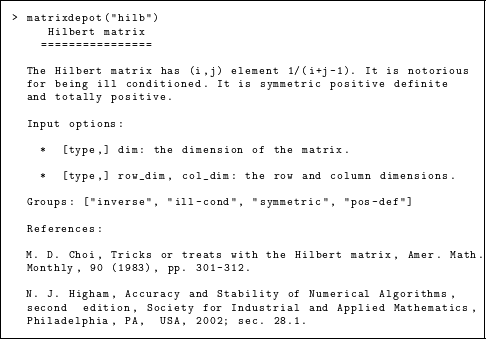Note that an optional first argument type can be given; it defaults to Float64. The string of equals signs on the third line in the output above is Markdown notation for a header. Julia interprets Markdown within documentation, though as we are using typewriter font for code examples here, we display the uninterpreted source. We generate a 4 × 6 Hilbert matrix with elements in the default double precision type and then in Rational type.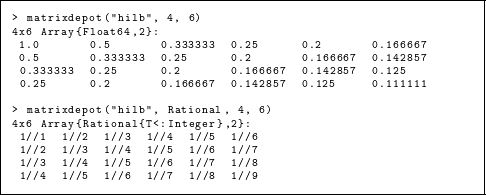A list of all the symmetric matrices in the collection is readily obtained.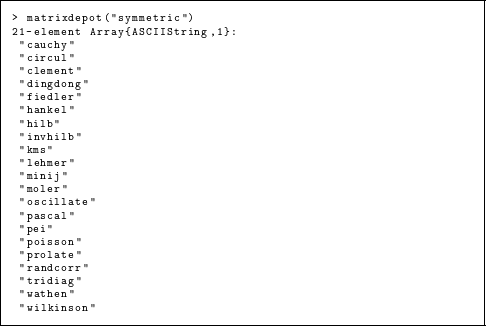Here, symmetric is one of several predefined groups, and multiple groups can be intersected. For example, the for loop below prints the smallest and largest eigenvalues of all the 4 × 4 matrices in Matrix Depot that are symmetric positive definite and (potentially) ill conditioned.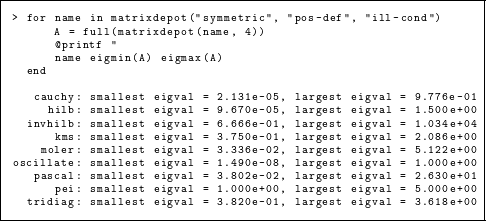Matrices can also be accessed by number within the alphabetical list of matrix names.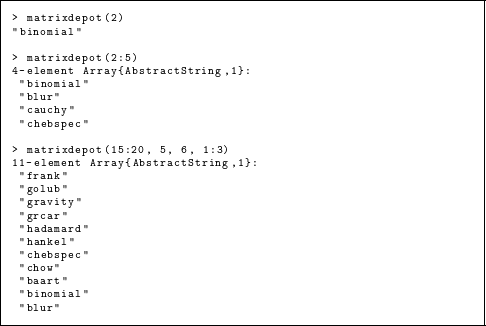Access by number provides a convenient way to run a test on subsets of matrices in the collection. However, the number assigned to a matrix may change if we include new matrices in the collection. In order to run tests in a way that is repeatable in the future it is best to group matrices into subsets using the macro @addgroup, which stores them by name. For example, the following command will group test matrices frank, golub, gravity, grcar, hadamard, hankel, chebspec, chow, baart, binomial, and blur into test1.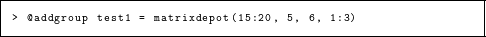After reloading the package, we can run tests on these matrices using group test1. Here we compute the 2-norms. Since blur (an image deblurring test problem) generates a sparse matrix and the matrix 2-norm is currently not implemented for sparse matrices in Julia, we use full to convert the matrix to dense format.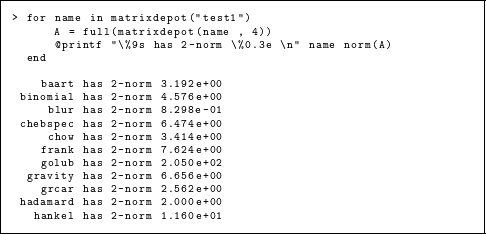To download the test matrix SNAP/web-Google from the University of Florida Sparse Matrix Collection (see ‘Matrix Data from External Sources’ for more details), we first download the data with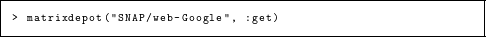and then generate the matrix with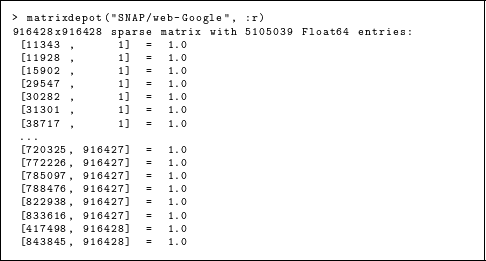Note that the omission marked “ ...” was in this case automatically done by Julia based on the height of the terminal window. Matrices loaded in this way are inserted into the list of available matrices, and assigned a number. After downloading further matrices HB/1138_bus, HB/494_bus, and Bova/rma10 the list of matrices is as follows.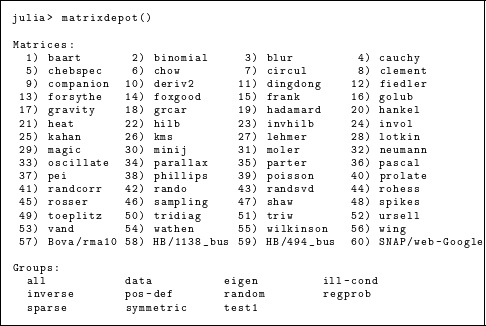## Package Design and Implementation

In this section we describe the design and implementation of Matrix Depot, focusing particularly on the novel aspects of exploitation of multiple dispatch, extensibility of the collection, and user-definable grouping of matrices.

### Exploiting multiple dispatch

Matrix Depot makes use of multiple dispatch in Julia, an object-oriented paradigm in which the selection of a function implementation is based on the types of each argument of the function. The generic function matrixdepot has eight different methods, where each method itself is a function that handles a specific case. This is neater and more convenient than writing eight “case” statements, as is necessary in many other languages.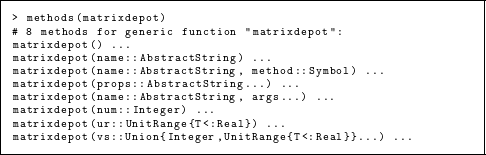For example, the following two functions are used for accessing matrices by number and range respectively, where matrix_name_list() returns a list of matrix names. The second function calls the first function in the inner loop.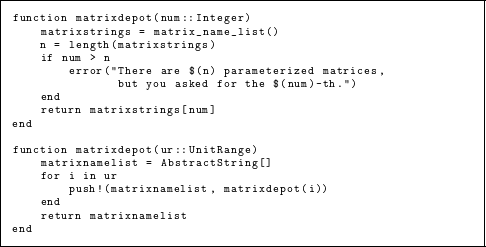As a result, matrixdepot is a versatile function that can be used for a variety of purposes, including returning matrix information and generating matrices from various input parameters.

In the following example we see how multiple dispatch handles different numbers and types of arguments for the Cauchy matrix.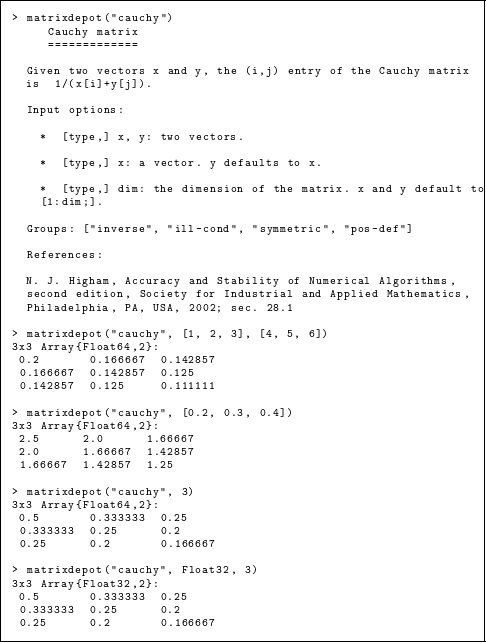Multiple dispatch is also exploited in programming the matrices. For example, the Hilbert matrix is implemented as

function hilb{T}(::Type{T}, m::Integer, n::Integer)
H = zeros(T, m, n)
for j = 1:n, i = 1:m
@inbounds H[i,j] = one(T)/ (i + j - one(T))
end
return H
end
hilb{T}(::Type{T}, n::Integer) = hilb(T, n, n)
hilb(args...) = hilb(Float64, args...)

The function hilb has three methods, which enable one to request, for example, hilb(4,2) for a 4 × 2 Hilbert matrix of type Float64, or simply (thanks to the final two lines) hilb(4) for a 4 × 4 Hilbert matrix of type Float64. The keyword @inbounds tells Julia to turn off bounds checking in the following expression, in order to speed up execution. Note that in Julia it is not necessary to vectorize code to achieve good performance (Bezanson et al., 2014).

All the matrices in Matrix Depot can be generated using the function call

matrixdepot("matrix_name", p1, p2, ...),

where matrix_name is the name of the test matrix, and p1, p2, …, are input arguments depending on matrix_name. The help comments for each matrix can be viewed by calling function matrixdepot(”matrix_name”). We can access the list of matrix names by number, range, or a mixture of numbers and range.

1. matrixdepot(i) returns the name of the ith matrix;

2. matrixdepot(i:j) returns the names of the ith to jth matrices, where i < j;

3. matrixdepot(i:j, k, m) returns the names of the ith, (i + 1)st, …, jth, kth, and mth matrices.

### Matrix representation

Matrix names in Matrix Depot are represented by Julia strings. For example, the Cauchy matrix is represented by ”cauchy”. Matrix names and matrix groups are stored as hash tables (Dict). In particular, there is a hash table matrixdict that maps each matrix name to its underlying function and a hash table matrixclass that maps each group to its members.

The majority of parametrized matrices are dense matrices of type Array{T,2}, where T is the element type of the matrix. Variables of the Array type are stored in column-major order. A few matrices are stored as sparse matrices (see also matrixdepot(”sparse”)), in the Compressed Sparse Column (CSC) format; these include neumann (a singular matrix from the discrete Neumann problem) and poisson (a block tridiagonal matrix from Poisson’s equation). Tridiagonal matrices are stored in the built-in Julia type Tridiagonal, which is defined as follows.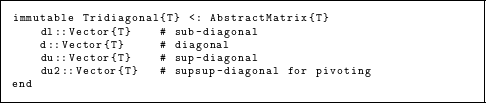### Matrix groups

A group is a subset of matrices in Matrix Depot. There are ten predefined groups, described in Table 1, most of which identify matrices with particular properties. Each group is represented by a string. For example, the group of random matrices is represented by ”random”. Matrices can be accessed by group names, as was illustrated in ‘A Taste of Matrix Depot.’

The macro @addgroup is used to add a new group of matrices to Matrix Depot and the macro @rmgroup removes an added group. All the predefined matrix groups are stored in the hash table matrixclass. The macro addgroup essentially adds a new key-value combination to the hash table usermatrixclass. Using a separate hash table prevents the user from contaminating the predefined matrix groups.

Being able to create groups is a useful feature for reproducible research (Donoho & Stodden, 2015). For example, if we have implemented algorithm alg01 and we used circul, minij, and grcar as test matrices for alg01, we could type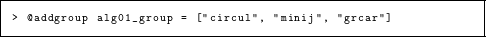This adds a new group to Matrix Depot (we need to reload the package to see the changes).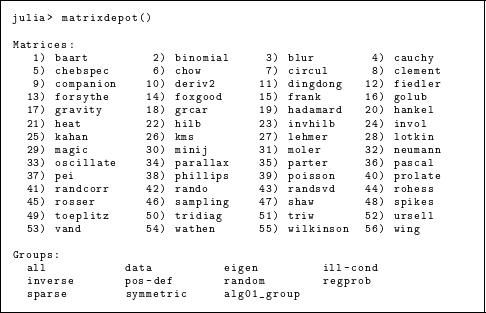We can then run alg01 on the test matrices by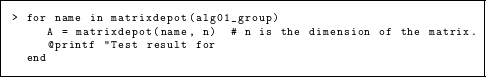### Adding new matrix generators

Generators are Julia functions that generate test matrices. When Matrix Depot is first loaded, a directory myMatrixDepot is created. It contains two files, group.jl and generator.jl, where group.jl is used for storing all the user-defined groups (see ‘Matrix Group’) and generator.jl is used for storing generator declarations.

Julia packages are simply Git repositories.2 The directory myMatrixDepot is untracked by Git, so any local changes to files in myMatrixDepot do not make the MatrixDepot package “dirty.” In particular, all the newly defined groups or matrix generators will not be affected when we upgrade to a new version of Matrix Depot. Matrix Depot automatically loads all Julia files in myMatrixDepot. This feature allows a user to simply drop generator files into myMatrixDepot without worrying about how to link them to Matrix Depot.

A new generator is declared using the syntax include_generator(FunctionName, ”fname”, f). This adds the new mapping ”fname”f to the hash table matrixdict, which we recall maps each matrix name to its underlying function. Matrix Depot will refer to function f using string ”fname” so that we can call function f by matrixdepot(”fname”...). The user is free to define new data types and return values of those types. Moreover, as with any Julia function, multiple values can be returned by listing them after the return statement.

For example, suppose we have the following Julia file rand.jl, which contains two generators randsym and randorth and we want to use them from Matrix Depot. The triple quotes in the file delimit the documentation for the functions.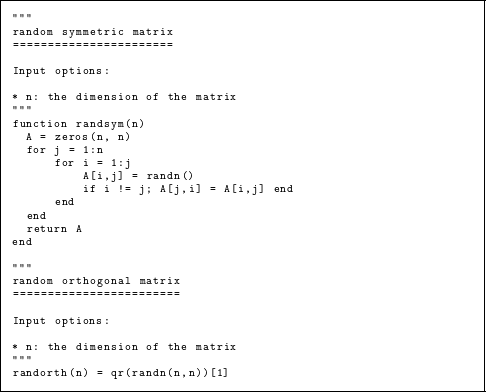We can copy the file rand.jl to the directory myMatrixDepot and add the following two lines to generator.jl.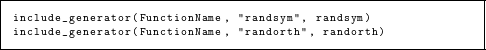This includes the functions randsym and randorth in Matrix Depot, as we can see by looking at the matrix list (the new entries are numbered 43 and 45).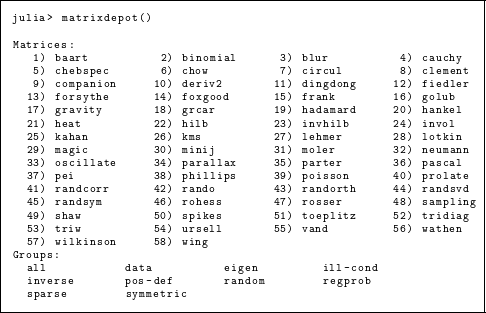The new generators can be used just like the built-in ones.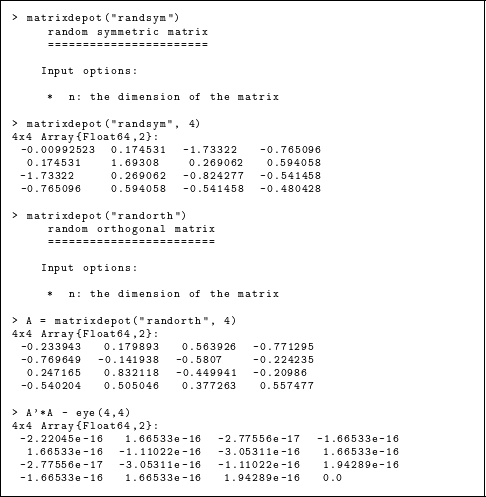We can also add group information with the function include_generator. The following lines are put in generator.jl.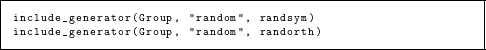This adds the functions randsym and randorth to the group random, as we can see with the following query (after reloading the package).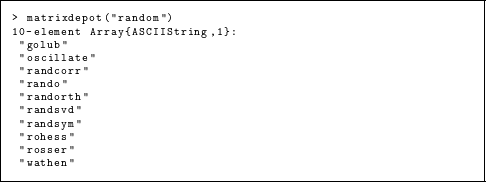### Documentation

The Matrix Depot documentation is created using the documentation generator Sphinx (http://sphinx-doc.org/) and is hosted at Read the Docs (http://matrixdepotjl.readthedocs.org). Its primary goals are to provide examples of usage of Matrix Depot and to give a brief summary of each matrix in the collection. Matrices are listed alphabetically with hyperlinks to the documentation for each matrix. Most parametrized matrices are presented with heat map plots, which are produced using the Winston package (https://github.com/nolta/Winston.jl), with the color range determined by the smallest and largest entries of the matrix. For example, Fig. 1 shows how the Wathen matrix is documented in Matrix Depot.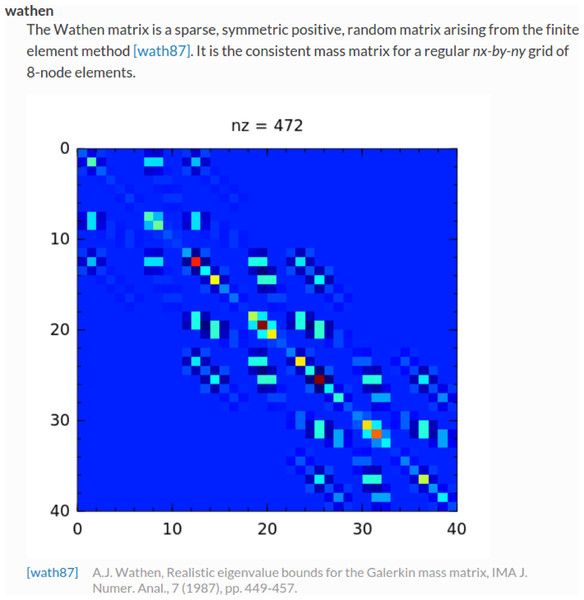Figure 1: Documentation for the Wathen matrix.   Download full-size image DOI: 10.7717/peerj-cs.58/fig-1

## The Matrices

We now describe the matrices that are provided with, or can be downloaded into, Matrix Depot.

### Parametrized matrices

In Matrix Depot v0.5.5, there are 58 parametrized matrices (including the regularization problems described in the next section), most of which originate from the Test Matrix Toolbox (Higham, 1995). All these matrices can be generated as matrixdepot(”matrix_name”, n), where n is the dimension of the matrix.

Many matrices can have more than one input parameter, and multiple dispatch provides a convenient mechanism for taking different actions for different argument types. For example, the tridiag function generates a tridiagonal matrix from vector arguments giving the subdiagonal, diagonal, and superdiagonal vectors, but a tridiagonal Toeplitz matrix can be obtained by supplying scalar arguments that specify the dimension of the matrix, the subdiagonal, the diagonal, and the superdiagonal. If a single, scalar argument n is supplied then an n-by- n tridiagonal Toeplitz matrix with subdiagonal and superdiagonal −1 and diagonal 2 is constructed. This matrix arises in applying central differences to a second derivative operator, and the inverse and the condition number are known explicitly (Higham, 2002, sec. 28.5).

Here is an example of the different usages of tridiag.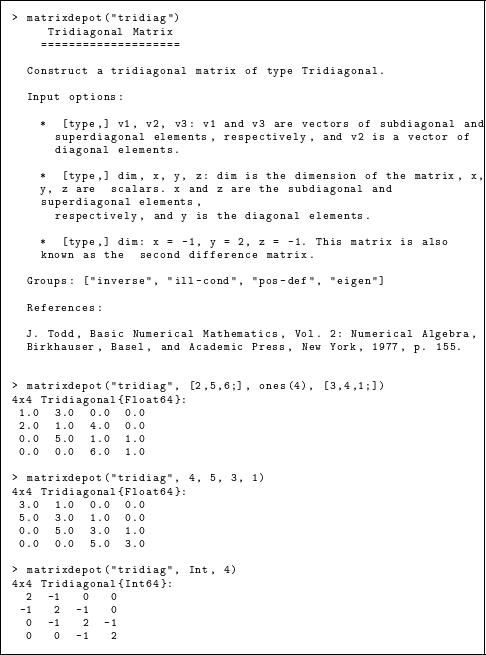#### Test problems for regularization methods

A mathematical problem is ill-posed if the solution is not unique or if an arbitrarily small perturbation of the data can cause an arbitrarily large change in the solution. Regularization methods are an important class of methods for dealing with such problems (Hansen, 1998; Hansen, 2010). One means of generating test problems for regularization methods is to discretize a given ill-posed problem.

Matrix Depot contains a group of regularization test problems derived from Hansen’s MATLAB Regularization Tools (Hansen, 1994; Hansen, 2007; Hansen, 2008) that are mostly discretizations of Fredholm integral equations of the first kind: ${\int }_{0}^{1}K\left(s,t\right)f\left(t\right)dt=g\left(s\right),\phantom{\rule{10.00002pt}{0ex}}0\le s\le 1.$ The regularization test problems form the group regprob.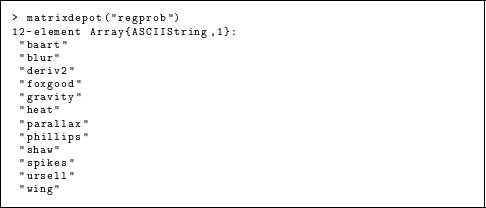Each problem is a linear system Ax = b where the matrix A and vectors x and b are obtained by discretization (using quadrature or the Galerkin method) of K, f, and g. By default, we generate only A, which is an ill-conditioned matrix. The whole test problem will be generated if the parameter matrixonly is set to false, and in this case the output has type RegProb, which is defined as

immutable RegProb{T}
A::AbstractMatrix{T} # matrix of interest
b::AbstractVector{T} # right-hand side
x::AbstractVector{T} # the solution to Ax = b
end

If r is a generated test problem, then r.A, r.b, and r.x are the matrix A and vectors x and b respectively. If the solution is not provided by the problem, the output is stored as type RegProbNoSolution, which is defined as

immutable RegProbNoSolution{T}
A::AbstractMatrix{T} # matrix of interest
b::AbstractVector{T} # right-hand side
end

For example, the test problem wing can be generated as follows.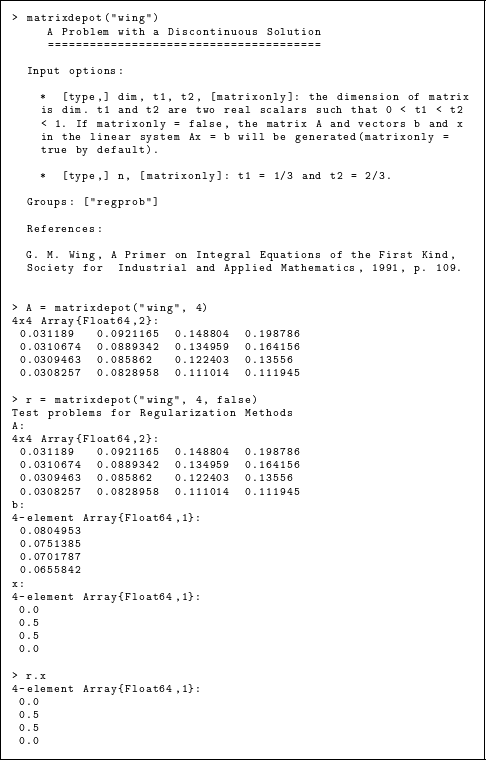### Matrix data from external sources

Matrix Depot provides access to matrices from Matrix Market (Boisvert et al., 1997) and the University of Florida Sparse Matrix Collection (Davis & Hu, 2011), both of which contain many matrices taken from applications. In particular, these sources contain many large, sparse matrices.

Matrix Market and the University of Florida Sparse Matrix Collection both categorize matrices by application domain and the problem source and both provide matrices in Matrix Market Format (Boisvert, Pozo & Remington, 1996). These similarities allow us to design a generic interface for both collections. The symbol :get (or :g) is used for downloading matrices from both collections and the symbol :read (or :r) is used for reading in matrices already downloaded. Downloaded matrix data is stored on disk in the Matrix Market format and when read into Julia is stored in the type SparseMatrixCSC.

MatrixDepot.update() downloads the matrix name data files from the two web servers.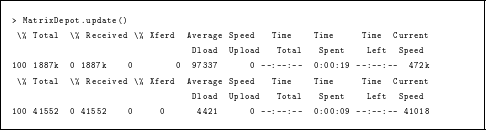The University of Florida Sparse Matrix Collection is divided into matrix groups and the group of a matrix forms part of the full name of the matrix (Davis & Hu, 2011). For example, the full name of the matrix 1138_bus in the Harwell-Boeing Collection is HB/1138_bus.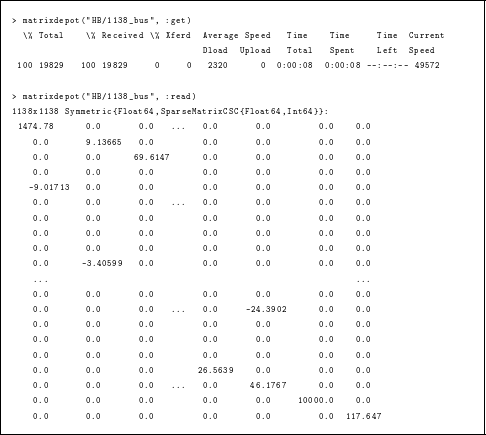Matrices from the University of Florida Sparse Matrix Collection are stored in MatrixDepot/data/uf and they are stored by group (to avoid duplicate names), i.e., one directory per group. Similarly, matrices from Matrix Market are stored in MatrixDepot/data/mm. Both directories are untracked by Git. Many matrices in the University of Florida Sparse Matrix Collection contain problem-specific metadata, all of which is downloaded. The metadata is accessed by setting the keyword argument meta to true. Then instead of returning the matrix, Matrix Depot will return the metadata (including the matrix) as a dictionary. For example, the IMDB movie database Pajek/IMDB has metadata related to actors and movies. The following command stores all the metadata of Pajek/IMDB in a variable r, where r[”IMDB”] is the matrix.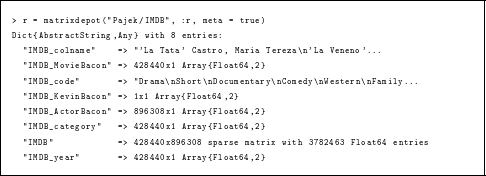We can download a whole group of matrices from the University of Florida sparse matrix collection using the command matrixdepot(”group name/*”, :get). The next example downloads all 67 matrices in the Gset group of matrices from random graphs (contributed by Y. Ye) then displays all the matrices in Matrix Depot, including the newly downloaded matrices.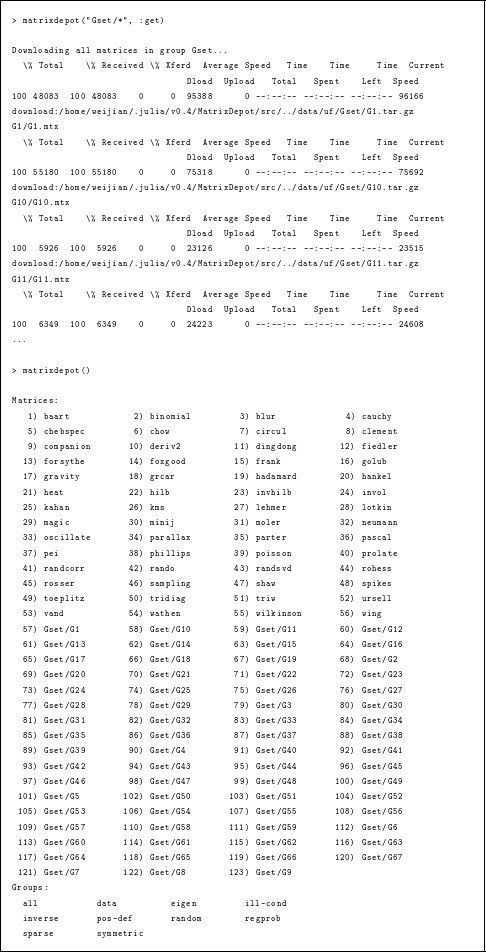The full name of a matrix in Matrix Market comprises three parts: the collection name, the set name, and the matrix name. For example, the full name of the matrix BCSSTK14 in the set BCSSTRUC2 from the Harwell-Boeing Collection is Harwell-Boeing/bcsstruc2/bcsstk14. Note that both set name and matrix name are in lower case.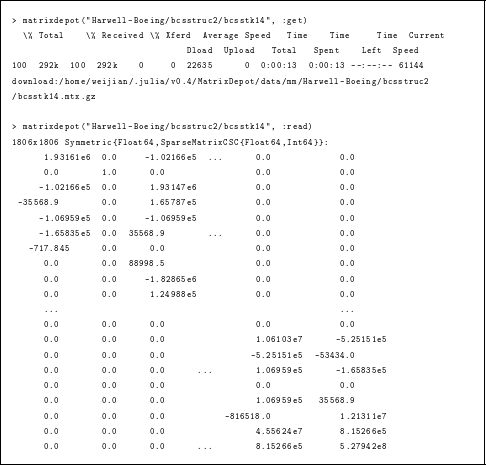We recommend downloading matrices from the University of Florida Sparse Matrix Collection when there is a choice, because almost every matrix from Matrix Market is included in it.

## Concluding Remarks

Matrix Depot follows in the footsteps of earlier collections of matrices. Its novelty is threefold. First, it is extensible by the user, and so can be adapted to the user’s needs. In doing so it facilitates experimentation, and in particular makes it easier to do reproducible research. Second, it combines several existing test matrix collections, namely Higham’s Test Matrix Toolbox, Hansen’s regularization problems, and the University of Florida Sparse Matrix Collection, in order to provide both parametrized test matrices and real-life sparse matrix data in a single framework. Third, it fully exploits the Julia language. It uses multiple dispatch to help provide a simple interface and, in particular, to allow matrices to be generated in any of the numeric data types supported by the language. Matrix Depot therefore anticipates the development of intrinsic support in Julia for computations with BigFloat and other data types.

Matrix Depot has been in development since 2014. It is an open source project (https://github.com/weijianzhang/MatrixDepot.jl) hosted on GitHub and is available under the MIT License. A first release was announced in December 2014. Matrix Depot v0.5.5 is the latest official release and consists of around 3, 000 lines of source code, with test coverage of 98.91% according to Codecov (https://codecov.io/). From GitHub traffic analytics, we learn that Matrix Depot has 40–70 unique downloads (unique cloners) every month. Matrix Depot also benefits the development of other Julia packages. LightGraphs (https://github.com/JuliaGraphs/LightGraphs.jl), an optimized graph package for Julia, for example, has embedded Matrix Depot as its database.

We built Matrix Depot to facilitate the development and testing of matrix (and other) algorithms in Julia. and we will continue to develop Matrix Depot by introducing new test matrices and integrating other test collections.

The University of Florida Sparse Matrix Collection is to be renamed as The SuiteSparse Matrix Collection.
Git is a free and open source distributed version control system.

## Acknowledgements

The authors are grateful to Jiahao Chen (MIT), Stefan Güttel (The University of Manchester), and Tim Davis (Texas A & M University) for suggestions, and to Per Christian Hansen for allowing us to incorporate problems from Regularization Tools.

## Additional Information and Declarations

### Competing Interests

Nicholas J. Higham is an Academic Edtor for PeerJ Computer Science.

### Author Contributions

Weijian Zhang conceived and designed the experiments, performed the experiments, analyzed the data, contributed reagents/materials/analysis tools, wrote the paper, prepared figures and/or tables, performed the computation work, reviewed drafts of the paper.

Nicholas J. Higham conceived and designed the experiments, analyzed the data, contributed reagents/materials/analysis tools, wrote the paper, performed the computation work, reviewed drafts of the paper.

### Data Availability

The following information was supplied regarding data availability:

Matrix Depot: https://github.com/weijianzhang/MatrixDepot.jl.

### Funding

The work of Higham was supported by European Research Council Advanced Grant MATFUN (267526) and Engineering and Physical Sciences Research Council grant EP/I01912X/1. The funders had no role in study design, data collection and analysis, decision to publish, or preparation of the manuscript.